Study P4 Mathematics Maths - Fractions - Geniebook# Fractions

Fractions are parts of a whole, for instance, \begin{align*} \frac{1} {2} \end{align*}. The three types of fractions are proper fractions, improper fractions and mixed numbers. A fraction is denoted as \begin{align*} \frac{x} {y} \end{align*}, where $$x$$ is the numerator and $$y$$ is the denominator.

1. Understanding improper fractions and mixed numbers
2. Express improper fractions as mixed numbers
3. Express mixed numbers as improper fractions

## 1. Understanding Improper Fractions And Mixed Numbers

• An improper fraction has a value equal to or greater than $$1$$
• The numerator is equal to or greater than the denominator.
• \begin{align*} \frac{4} {4} \end{align*}, \begin{align*} \frac{5} {4} \end{align*} and \begin{align*} \frac{10} {3} \end{align*} are examples of  improper fractions.
• A mixed number is made up of a whole number and a fraction.
• \begin{align*} 3\frac{1} {4} \end{align*}, \begin{align*} 1\frac{5} {7} \end{align*} and \begin{align*} 20\frac{2} {9} \end{align*} are examples of mixed numbers.
• An improper fraction can be expressed as a mixed number.

Example: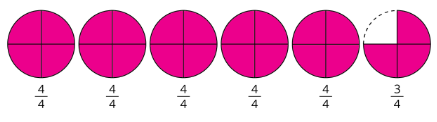\begin{align*} \frac{23} {4} \end{align*} is an improper fraction.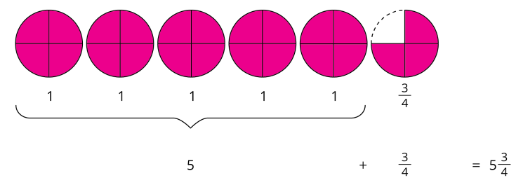\begin{align*} 5\frac{3} {4} \end{align*} is a mixed number.

Question 1:

Which of the following is an improper fraction?

1. \begin{align*} \frac{3} {4} \\ \end{align*}
2. \begin{align*} \frac{7} {8} \end{align*}
3. \begin{align*} \frac{5} {4} \end{align*}
4. \begin{align*} 5\frac{1} {5} \end{align*}

(3) \begin{align*} \frac{5} {4} \end{align*}

Question 2:

Which of the following is not an improper fraction?

​​​​​​

1. \begin{align*} \frac{8} {7} \end{align*}
2. \begin{align*} \frac{15} {3} \end{align*}
3. \begin{align*} \frac{9} {9} \end{align*}
4. \begin{align*} \frac{7} {10} \end{align*}

(4) \begin{align*} \frac{7} {10} \end{align*}

Question 3:

Which of the following is a mixed number?

1. \begin{align*} \frac{9} {7} \end{align*}
2. \begin{align*} 3\frac{6} {13} \end{align*}
3. \begin{align*} \frac{8} {8} \end{align*}
4. \begin{align*} \frac{1} {10} \end{align*}

(2) \begin{align*} 3\frac{6} {13} \end{align*}

Question 4:

What mixed number does the following represent?1. \begin{align*} \frac{9} {4} \end{align*}
2. \begin{align*} \frac{63} {4} \end{align*}
3. \begin{align*} 6\frac{3} {4} \end{align*}
4. \begin{align*} 7\frac{3} {4} \end{align*}

Solution:(3) \begin{align*} 6\frac{3} {4} \end{align*}

Question 5:

What improper fraction does the following represent?Solution:\begin{align*} \frac{8} {8}+\frac{8} {8}+\frac{5} {8} = \frac{21} {8} \end{align*}

\begin{align*} \frac{21} {8} \end{align*}

## 2. Express Improper Fractions As Mixed Numbers

There are 2 methods to express improper fractions as mixed numbers:

1. Expressing to the greatest possible number of wholes
2. Long Division

Question 1:

How many sevenths are there in 8 wholes?

Solution:

\begin{align*} ​1 \;\text{whole} &= \frac {7}{7} \\ \\ & = 7 \;\text{sevenths} \\ \\ \\ ​ 8 \;\text{wholes} &= \frac {56}{7} \\ \\ &= 56 \;\text{sevenths} ​ \end{align*}

$$56$$

Question 2:

How many fifths are there in 3 wholes?

Solution:

\begin{align*} ​1 \;\text{whole} &= \frac {5}{5} \\ \\ & = 5 \;\text{fifths} \\ \\ \\ ​ 3 \;\text{wholes} &= \frac {15}{5} \\ \\ &= 15 \;\text{fifths} ​ \end{align*}

$$15$$

Question 3:

Express \begin{align*} \frac{28} {5} \end{align*} as a mixed number.

Solution:

\begin{align*} \frac{28} {5} &=\frac{25} {5} +\frac{3} {5} \\ \\ &= 5+\frac{3} {5} \\ \\ &= 5\frac{3} {5} \end{align*}

or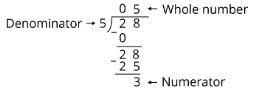\begin{align*} 5\frac{3} {5} \end{align*}

Question 4:

Express \begin{align*} \frac{26} {3} \end{align*} as a mixed number.

Solution:

\begin{align*} \frac{26} {3} &=\frac{24} {3} +\frac{2} {3} \\ \\ &= 8+\frac{2} {3} \\ \\ &= 8\frac{2} {3} \end{align*}

or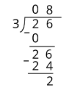\begin{align*} 8\frac{2} {3} \end{align*}

Question 5:

Convert \begin{align*} \frac{19} {3} \end{align*} to a mixed number.

Solution:

\begin{align*} \frac{19} {3} &=\frac{18} {3} +\frac{1} {3} \\ \\ &= 6+\frac{1} {3} \\ \\ &= 6\frac{1} {3} \end{align*}

or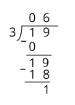\begin{align*} 6\frac{1} {3} \end{align*}

Question 6:

Convert \begin{align*} \frac{26} {5} \end{align*} to a mixed number.

Solution:

\begin{align*} \frac{26} {5} &=\frac{25} {5} +\frac{1} {5} \\ \\ &= 5+\frac{1} {5} \\ \\ &= 5\frac{1} {5} \end{align*}

or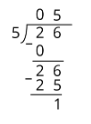\begin{align*} 5\frac{1} {5} \end{align*}

## 3. Express Mixed Numbers As Improper Fractions

We can express mixed numbers as improper fractions by expressing the wholes as improper fractions and then adding the remaining fraction.

Example:

\begin{align*} 3\frac{2} {5} &=3 +\frac{2} {5} \\ \\ &= \frac{15} {5}+\frac{2} {5} \\ \\ &= \frac{17} {5} \end{align*}

The short-cut method is as shown.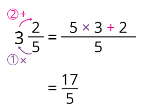Question 1:

Convert \begin{align*} 8\frac{3} {8} \end{align*} to an improper fraction.

1. \begin{align*} \frac{8} {67} \end{align*}
2. \begin{align*} \frac{24} {8} \end{align*}
3. \begin{align*} \frac{67} {8} \end{align*}
4. \begin{align*} \frac{83} {8} \end{align*}

Solution:

\begin{align*} 8\frac{3} {8} &=8 +\frac{3} {8} \\ \\ &= \frac{64} {8}+\frac{3} {8} \\ \\ &= \frac{67} {8} \end{align*}

The short-cut method is as shown.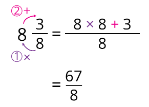(3) \begin{align*} \frac{67} {8} \end{align*}

Question 2:

Convert \begin{align*} 6\frac{4} {7} \end{align*} to an improper fraction.

Solution:

\begin{align*} 6\frac{4} {7} &=6 +\frac{4} {7} \\ \\ &= \frac{42} {7}+\frac{4} {7} \\ \\ &= \frac{46} {7} \end{align*}

The short-cut method is as shown.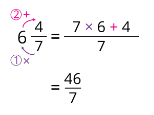\begin{align*} \frac{46} {7} \end{align*}

Question 3:

Convert \begin{align*} 13\frac{1} {6} \end{align*} to an improper fraction.

Solution:

\begin{align*} 13\frac{1} {6} &=13 +\frac{1} {6} \\ \\ &= \frac{79} {6} \end{align*}

The short-cut method is as shown.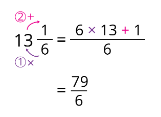\begin{align*} \frac{79} {6} \end{align*}

Question 4:

How many thirds are there in \begin{align*} 2\frac{1} {3} \end{align*}?

1. \begin{align*} \frac{21} {3} \end{align*}
2. \begin{align*} \frac{7} {3} \end{align*}
3. \begin{align*} 21 \end{align*}
4. \begin{align*} 7 \end{align*}

Solution:

Express the mixed number as an improper fraction with denominator 3.

\begin{align*} 2\frac{1} {3} &=2 +\frac{1} {3} \\ \\ &= \frac{6} {3}+\frac{1} {3} \\ \\ &= \frac{7} {3} \\ \\ &= 7 \;\text{third} \end{align*}

The short-cut method is as shown.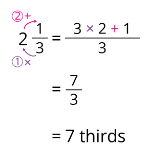(4) $$7$$

Question 5:

How many halves are there in \begin{align*} 5\frac{1} {2} \end{align*}?

Solution:

Express the mixed number as an improper fraction with denominator $$2$$.

\begin{align*} 5\frac{1} {2} &=5 +\frac{1} {2} \\ \\ &= \frac{10} {2}+\frac{1} {2} \\ \\ &= \frac{11} {2} \\ \\ &= 11 \;\text{halves} \end{align*}

The short-cut method is as shown.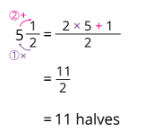\begin{align*} 11 \end{align*}

Question 6:

How many eighths are there in \begin{align*} 6\frac{3} {4} \end{align*}?

Solution:

Express the mixed number to an improper fraction with denominator \begin{align*} 8 \end{align*}.

\begin{align*} 6\frac{3} {4} &= \frac{27} {4} \\ \\ &= \frac{54} {8} \\ \\ &= 54 \; \text{eights} \end{align*}

The short-cut method is as shown.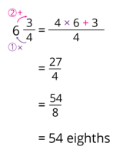\begin{align*} 54 \end{align*}

Continue Learning
Multiplication Whole Numbers
Multiplication And Division Decimals
Model Drawing Strategy Division
Fractions Factors And Multiples
Area And Perimeter 1 Line Graphs
TimePrimaryPrimary 1Primary 2Primary 3Primary 4EnglishMaths
Multiplication
Whole Numbers
Line Graphs
Time
Multiplication And Division
Decimals
Model Drawing Strategy
Division
Fractions
Factors And Multiples
Area And Perimeter 1SciencePrimary 5Primary 6SecondarySecondary 1Secondary 2Secondary 3Secondary 4